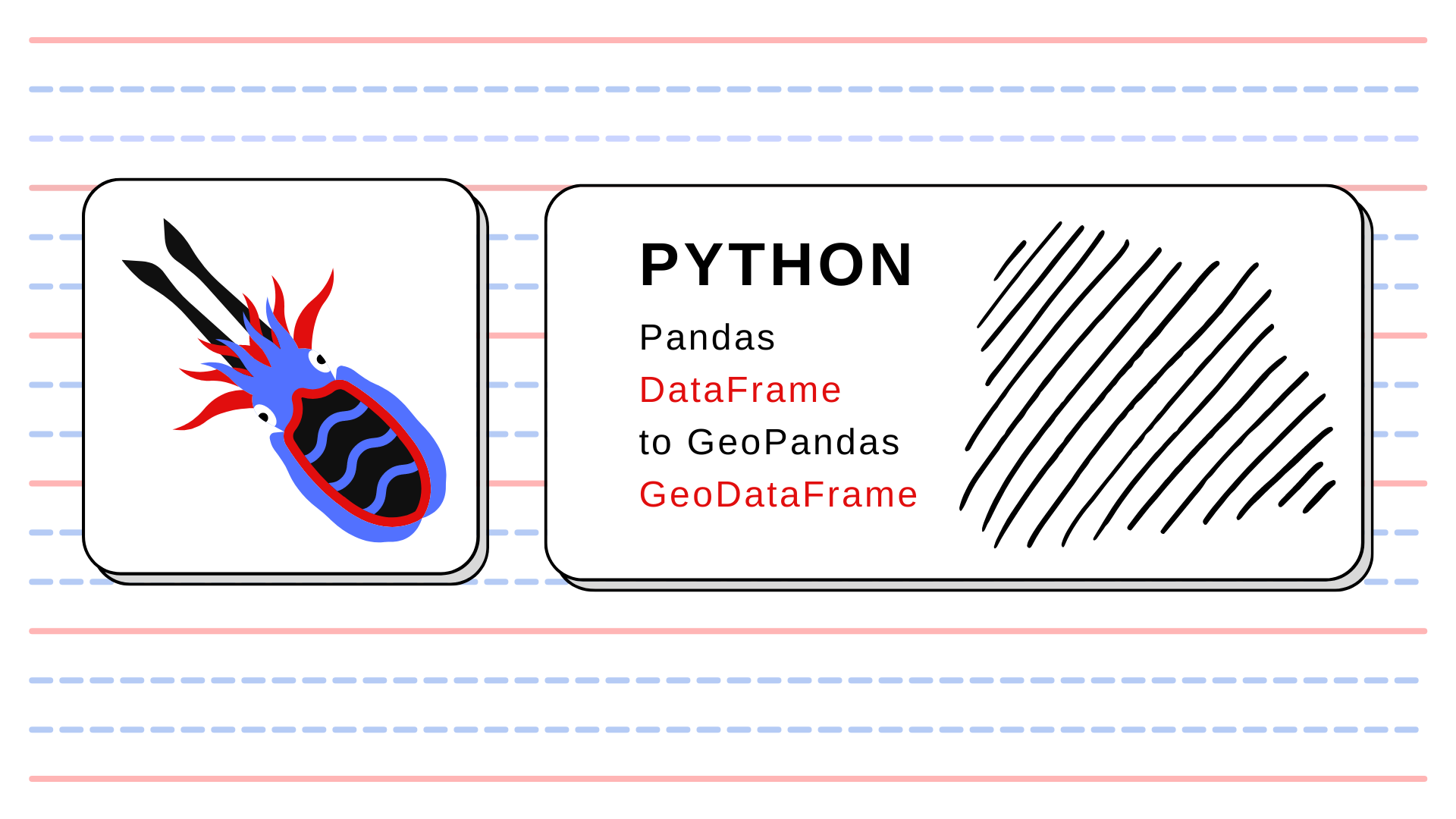Top
Sp.4ML > pandas  > Toolbox: Pandas DataFrame into GeoPandas GeoDataFrame## Toolbox: Pandas DataFrame into GeoPandas GeoDataFrame

Have you ever had problems visualizing spatial data read from a csv file? Is your spatial data stored in flat tables with lon / lat columns? I encountered those problems, that’s why I use a simple to transform quickly transform DataFrame into GeoDataFrame:

import geopandas as gpd

def df2gdf(df, lon_col='x', lat_col='y', epsg=4326, crs=None):
"""
Function transforms DataFrame into GeoDataFrame.

Parameters
----------
df : pandas DataFrame

lon_col : str, default = 'x'
Longitude column name.

lat_col : str, default = 'y'
Latitude column name.

epsg : Union[int, str], default = 4326
EPSG number of projection.

crs : str, default = None
Coordinate Reference System of data.

Returns
-------
gdf : GeoPandas GeoDataFrame
GeoDataFrame with set geometry column ('geometry'), CRS, and all columns from the passed DataFrame.

"""

gdf = gpd.GeoDataFrame(df)
gdf['geometry'] = gpd.points_from_xy(x=df[lon_col], y=df[lat_col])
gdf.geometry = gdf['geometry']

if crs is None:
gdf.set_crs(epsg=epsg, inplace=True)
else:
gdf.set_crs(crs=crs, inplace=True)

return gdf



The basic differences between DataFrame and GeoDataFrame are:

• column with the geometry data types,
• projection (coordinate reference system).

The function df2gdf() takes DataFrame, longitude column, latitude column, and crs or epsg to set a valid projection of the output structure. If you have provided DataFrame with a column that stores geometries (Point, Line, Polygon) then, the line gdf['geometry'] = gpd.points_from_xy(x=df[lon_col], y=df[lat_col]) is not required here. However, I assume that when we read a plain table, then geometries are provided as x and y columns, or lon and lat columns. In this scenario, we must transform a tuple of floats.##### Szymon
Subscribe
Notify of